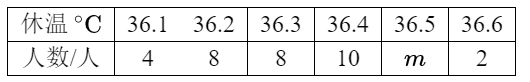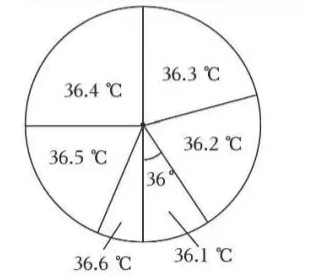$\text{A.}$ 这个班有 40 名学生 $\text{B.}$ $m=8$ $\text{C.}$ 这些体温的众数是 8 $\text{D.}$ 这些体温的中位数是 36.35

C

#### 解析：

【解答】解：由扇形统计图可知, 体温为 $36.1^{\circ} C$ 的学生人数所占百分比为 $\frac{36}{360} \times 100 \%$ $=10 \%$